Type
Quiz
Book Title
Fundamentals of Corporate Finance Standard Edition 9th Edition
ISBN 13
978-0073382395

### 978-0073382395 Chapter 6 Questions and Problems 1-10

April 3, 2019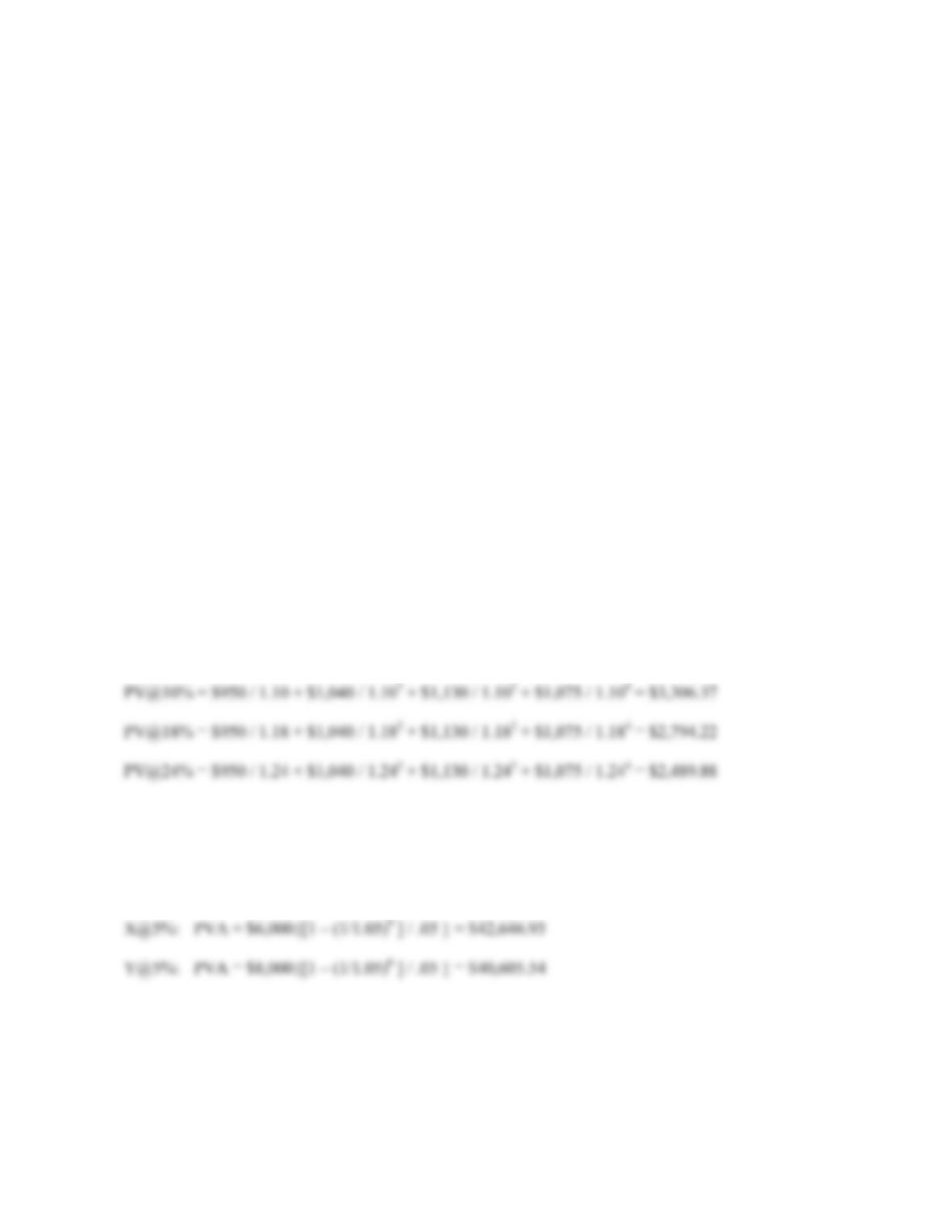CHAPTER 6 B-69
10. In general, viatical settlements are ethical. In the case of a viatical settlement, it is simply an exchange of
cash today for payment in the future, although the payment depends on the death of the seller. The
purchaser of the life insurance policy is bearing the risk that the insured individual will live longer than
expected. Although viatical settlements are ethical, they may not be the best choice for an individual. In a
Business Week article (October 31, 2005), options were examined for a 72 year old male with a life
expectancy of 8 years and a \$1 million dollar life insurance policy with an annual premium of \$37,000. The
four options were: 1) Cash the policy today for \$100,000. 2) Sell the policy in a viatical settlement for
\$275,000. 3) Reduce the death benefit to \$375,000, which would keep the policy in force for 12 years
without premium payments. 4) Stop paying premiums and don’t reduce the death benefit. This will run the
cash value of the policy to zero in 5 years, but the viatical settlement would be worth \$475,000 at that time.
If he died within 5 years, the beneficiaries would receive \$1 million. Ultimately, the decision rests on the
individual on what they perceive as best for themselves. The values that will affect the value of the viatical
settlement are the discount rate, the face value of the policy, and the health of the individual selling the
policy.
Solutions to Questions and Problems
NOTE: All end of chapter problems were solved using a spreadsheet. Many problems require multiple steps.
Due to space and readability constraints, when these intermediate steps are included in this solutions manual,
rounding may appear to have occurred. However, the final answer for each problem is found without rounding
during any step in the problem.
Basic
1. To solve this problem, we must find the PV of each cash flow and add them. To find the PV of a lump sum,
we use:
PV = FV / (1 + r)t
2. To find the PVA, we use the equation:
PVA = C({1 – [1/(1 + r)]t } / r )
At a 5 percent interest rate: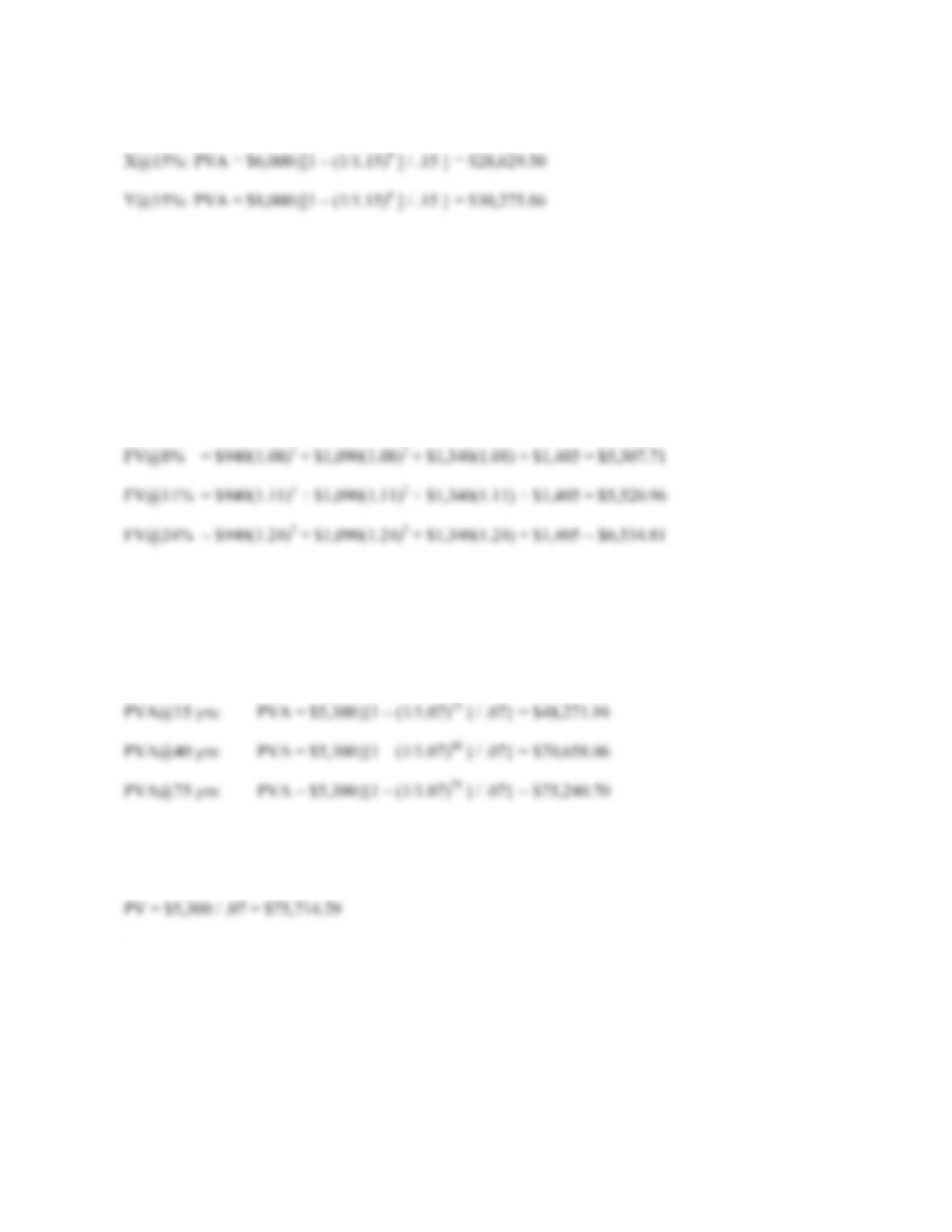B-70 SOLUTIONS
And at a 15 percent interest rate:
Notice that the PV of cash flow X has a greater PV at a 5 percent interest rate, but a lower PV at a 15
percent interest rate. The reason is that X has greater total cash flows. At a lower interest rate, the total cash
flow is more important since the cost of waiting (the interest rate) is not as great. At a higher interest rate,
Y is more valuable since it has larger cash flows. At the higher interest rate, these bigger cash flows early
are more important since the cost of waiting (the interest rate) is so much greater.
3. To solve this problem, we must find the FV of each cash flow and add them. To find the FV of a lump sum,
we use:
FV = PV(1 + r)t
Notice we are finding the value at Year 4, the cash flow at Year 4 is simply added to the FV of the other
cash flows. In other words, we do not need to compound this cash flow.
4. To find the PVA, we use the equation:
PVA = C({1 – [1/(1 + r)]t } / r )
To find the PV of a perpetuity, we use the equation:
PV = C / r
Notice that as the length of the annuity payments increases, the present value of the annuity approaches the
present value of the perpetuity. The present value of the 75 year annuity and the present value of the
perpetuity imply that the value today of all perpetuity payments beyond 75 years is only \$473.59.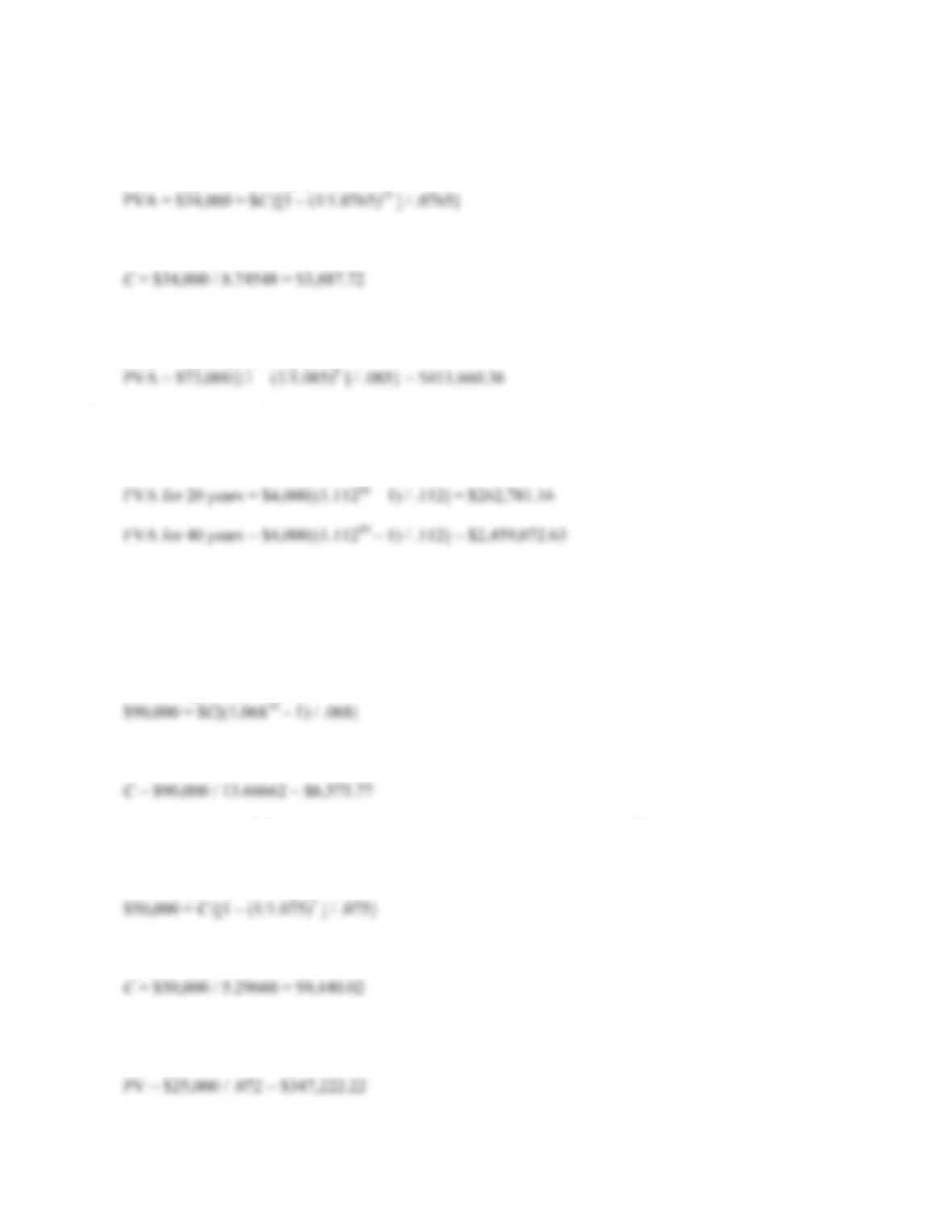CHAPTER 6 B-71
5. Here we have the PVA, the length of the annuity, and the interest rate. We want to calculate the annuity
payment. Using the PVA equation:
PVA = C({1 – [1/(1 + r)]t } / r )
We can now solve this equation for the annuity payment. Doing so, we get:
6. To find the PVA, we use the equation:
PVA = C({1 – [1/(1 + r)]t } / r )
7. Here we need to find the FVA. The equation to find the FVA is:
FVA = C{[(1 + r)t – 1] / r}
Notice that because of exponential growth, doubling the number of periods does not merely double the
FVA.
8. Here we have the FVA, the length of the annuity, and the interest rate. We want to calculate the annuity
payment. Using the FVA equation:
FVA = C{[(1 + r)t – 1] / r}
We can now solve this equation for the annuity payment. Doing so, we get:
9. Here we have the PVA, the length of the annuity, and the interest rate. We want to calculate the annuity
payment. Using the PVA equation:
PVA = C({1 – [1/(1 + r)]t } / r)
We can now solve this equation for the annuity payment. Doing so, we get:
10. This cash flow is a perpetuity. To find the PV of a perpetuity, we use the equation:
PV = C / r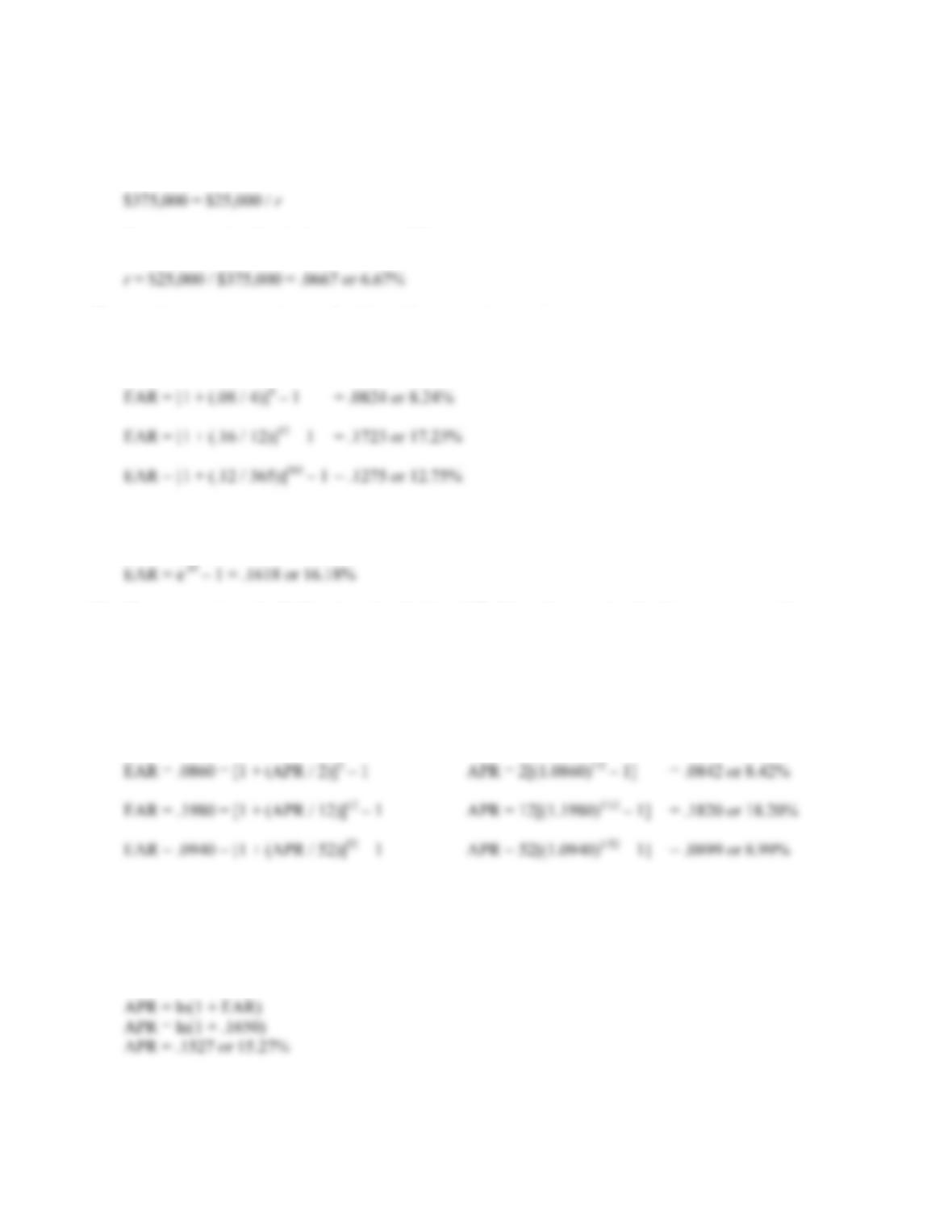B-72 SOLUTIONS
11. Here we need to find the interest rate that equates the perpetuity cash flows with the PV of the cash flows.
Using the PV of a perpetuity equation:
PV = C / r
We can now solve for the interest rate as follows:
12. For discrete compounding, to find the EAR, we use the equation:
EAR = [1 + (APR / m)]m – 1
To find the EAR with continuous compounding, we use the equation:
EAR = eq – 1
13. Here we are given the EAR and need to find the APR. Using the equation for discrete compounding:
EAR = [1 + (APR / m)]m – 1
We can now solve for the APR. Doing so, we get:
APR = m[(1 + EAR)1/m – 1]
Solving the continuous compounding EAR equation:
EAR = eq – 1
We get: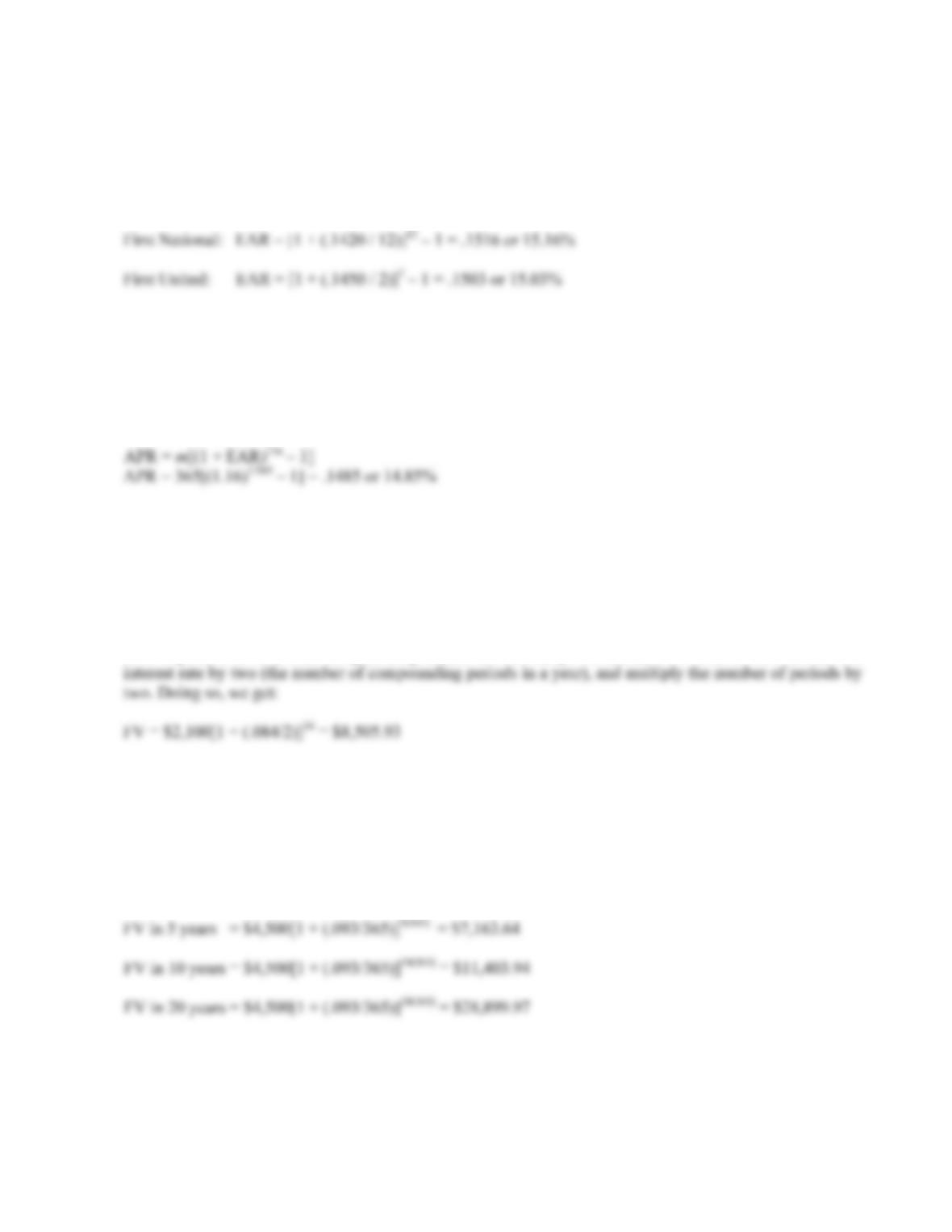CHAPTER 6 B-73
14. For discrete compounding, to find the EAR, we use the equation:
EAR = [1 + (APR / m)]m – 1
So, for each bank, the EAR is:
Notice that the higher APR does not necessarily mean the higher EAR. The number of compounding
periods within a year will also affect the EAR.
15. The reported rate is the APR, so we need to convert the EAR to an APR as follows:
EAR = [1 + (APR / m)]m – 1
This is deceptive because the borrower is actually paying annualized interest of 16% per year, not the
14.85% reported on the loan contract.
16. For this problem, we simply need to find the FV of a lump sum using the equation:
FV = PV(1 + r)t
It is important to note that compounding occurs semiannually. To account for this, we will divide the
17. For this problem, we simply need to find the FV of a lump sum using the equation:
FV = PV(1 + r)t
It is important to note that compounding occurs daily. To account for this, we will divide the interest rate
by 365 (the number of days in a year, ignoring leap year), and multiply the number of periods by 365.
Doing so, we get: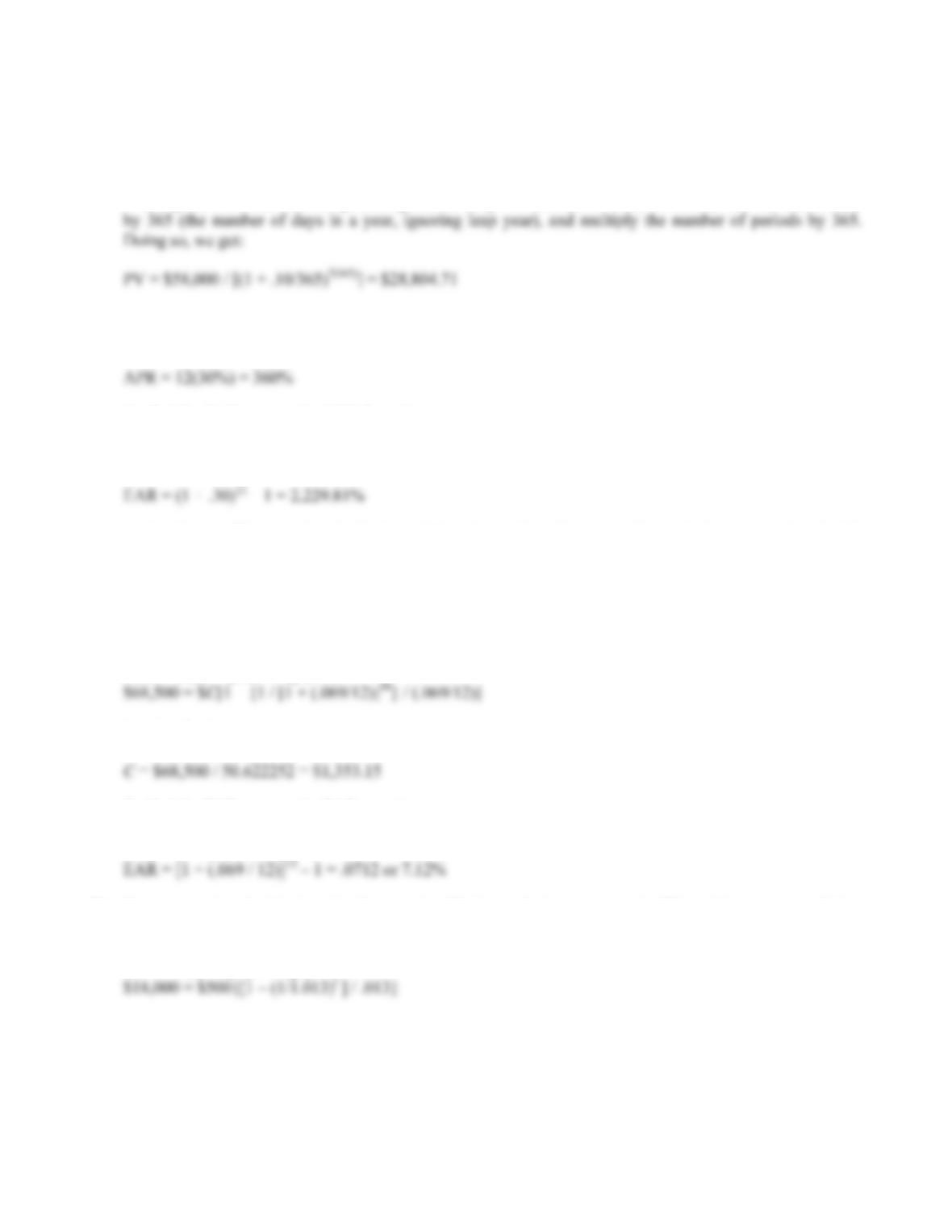B-74 SOLUTIONS
18. For this problem, we simply need to find the PV of a lump sum using the equation:
PV = FV / (1 + r)t
It is important to note that compounding occurs daily. To account for this, we will divide the interest rate
19. The APR is simply the interest rate per period times the number of periods in a year. In this case, the
interest rate is 30 percent per month, and there are 12 months in a year, so we get:
To find the EAR, we use the EAR formula:
EAR = [1 + (APR / m)]m – 1
Notice that we didn’t need to divide the APR by the number of compounding periods per year. We do this
division to get the interest rate per period, but in this problem we are already given the interest rate per
period.
20. We first need to find the annuity payment. We have the PVA, the length of the annuity, and the interest
rate. Using the PVA equation:
PVA = C({1 – [1/(1 + r)]t } / r)
Solving for the payment, we get:
To find the EAR, we use the EAR equation:
EAR = [1 + (APR / m)]m – 1
21. Here we need to find the length of an annuity. We know the interest rate, the PV, and the payments. Using
the PVA equation:
PVA = C({1 – [1/(1 + r)]t } / r)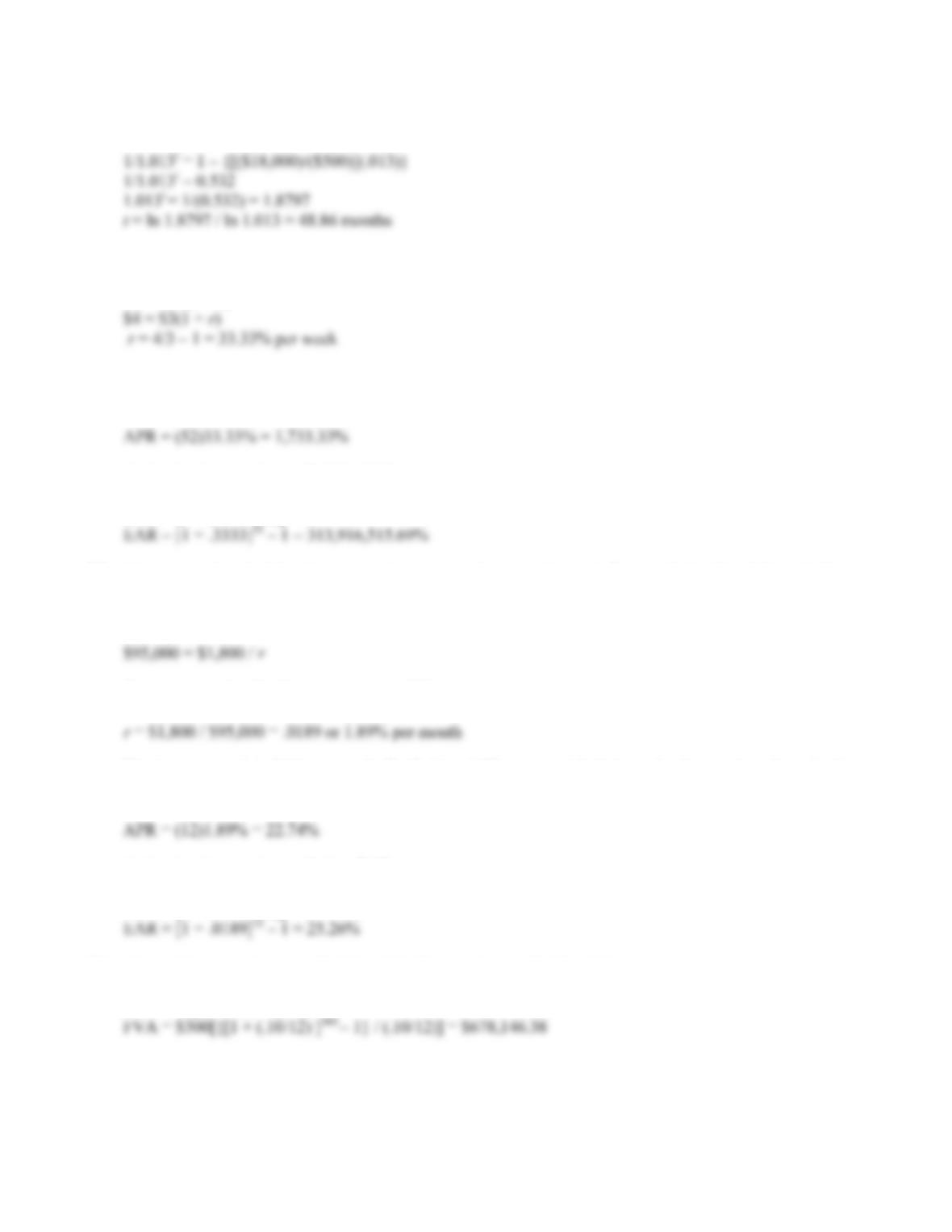CHAPTER 6 B-75
Now we solve for t:
22. Here we are trying to find the interest rate when we know the PV and FV. Using the FV equation:
FV = PV(1 + r)
The interest rate is 33.33% per week. To find the APR, we multiply this rate by the number of weeks in a
year, so:
And using the equation to find the EAR:
EAR = [1 + (APR / m)]m – 1
23. Here we need to find the interest rate that equates the perpetuity cash flows with the PV of the cash flows.
Using the PV of a perpetuity equation:
PV = C / r
We can now solve for the interest rate as follows:
The interest rate is 1.89% per month. To find the APR, we multiply this rate by the number of months in a
year, so:
And using the equation to find an EAR:
EAR = [1 + (APR / m)]m – 1
24. This problem requires us to find the FVA. The equation to find the FVA is:
FVA = C{[(1 + r)t – 1] / r}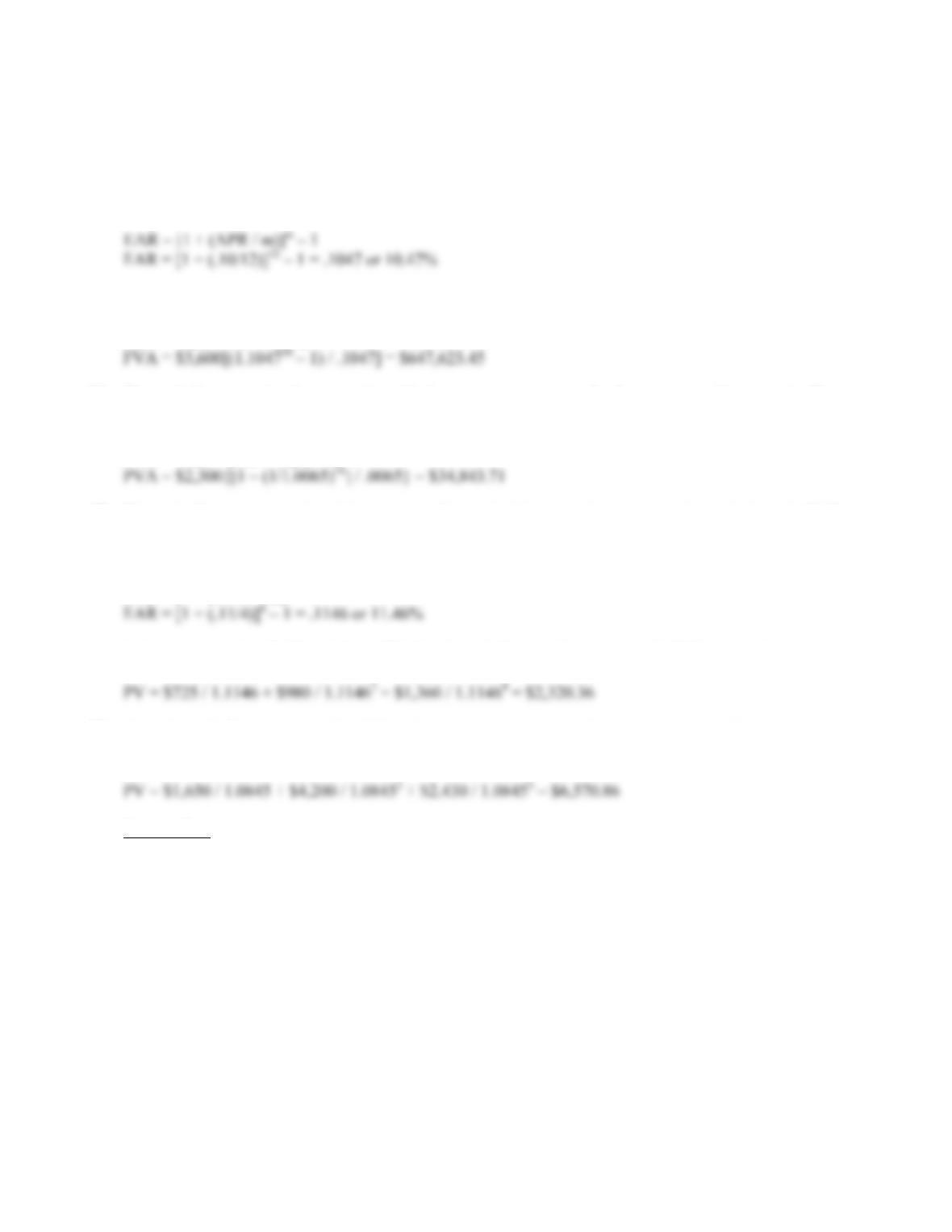B-76 SOLUTIONS
25. In the previous problem, the cash flows are monthly and the compounding period is monthly. This
assumption still holds. Since the cash flows are annual, we need to use the EAR to calculate the future
value of annual cash flows. It is important to remember that you have to make sure the compounding
periods of the interest rate is the same as the timing of the cash flows. In this case, we have annual cash
flows, so we need the EAR since it is the true annual interest rate you will earn. So, finding the EAR:
Using the FVA equation, we get:
FVA = C{[(1 + r)t – 1] / r}
26. The cash flows are simply an annuity with four payments per year for four years, or 16 payments. We can
use the PVA equation:
PVA = C({1 – [1/(1 + r)]t } / r)
27. The cash flows are annual and the compounding period is quarterly, so we need to calculate the EAR to
make the interest rate comparable with the timing of the cash flows. Using the equation for the EAR, we
get:
EAR = [1 + (APR / m)]m – 1
And now we use the EAR to find the PV of each cash flow as a lump sum and add them together:
28. Here the cash flows are annual and the given interest rate is annual, so we can use the interest rate given.
We simply find the PV of each cash flow and add them together.
Intermediate
29. The total interest paid by First Simple Bank is the interest rate per period times the number of periods. In
other words, the interest by First Simple Bank paid over 10 years will be:
.07(10) = .7
First Complex Bank pays compound interest, so the interest paid by this bank will be the FV factor of \$1,
or:
(1 + r)10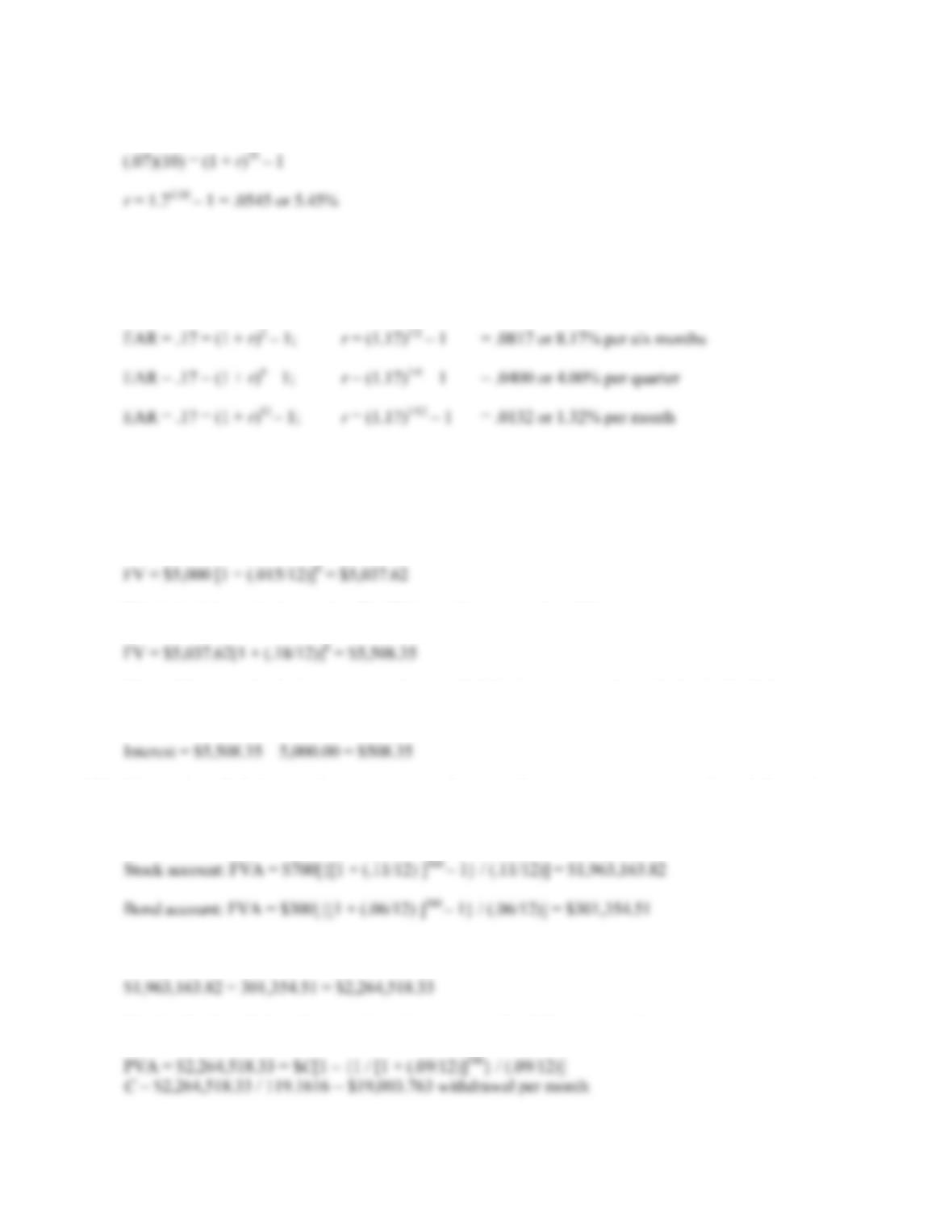CHAPTER 6 B-77
Setting the two equal, we get:
30. Here we need to convert an EAR into interest rates for different compounding periods. Using the equation
for the EAR, we get:
EAR = [1 + (APR / m)]m – 1
Notice that the effective six month rate is not twice the effective quarterly rate because of the effect of
compounding.
31. Here we need to find the FV of a lump sum, with a changing interest rate. We must do this problem in two
parts. After the first six months, the balance will be:
This is the balance in six months. The FV in another six months will be:
The problem asks for the interest accrued, so, to find the interest, we subtract the beginning balance
from the FV. The interest accrued is:
32. We need to find the annuity payment in retirement. Our retirement savings ends and the retirement
withdrawals begin, so the PV of the retirement withdrawals will be the FV of the retirement savings. So,
we find the FV of the stock account and the FV of the bond account and add the two FVs.
So, the total amount saved at retirement is:
Solving for the withdrawal amount in retirement using the PVA equation gives us: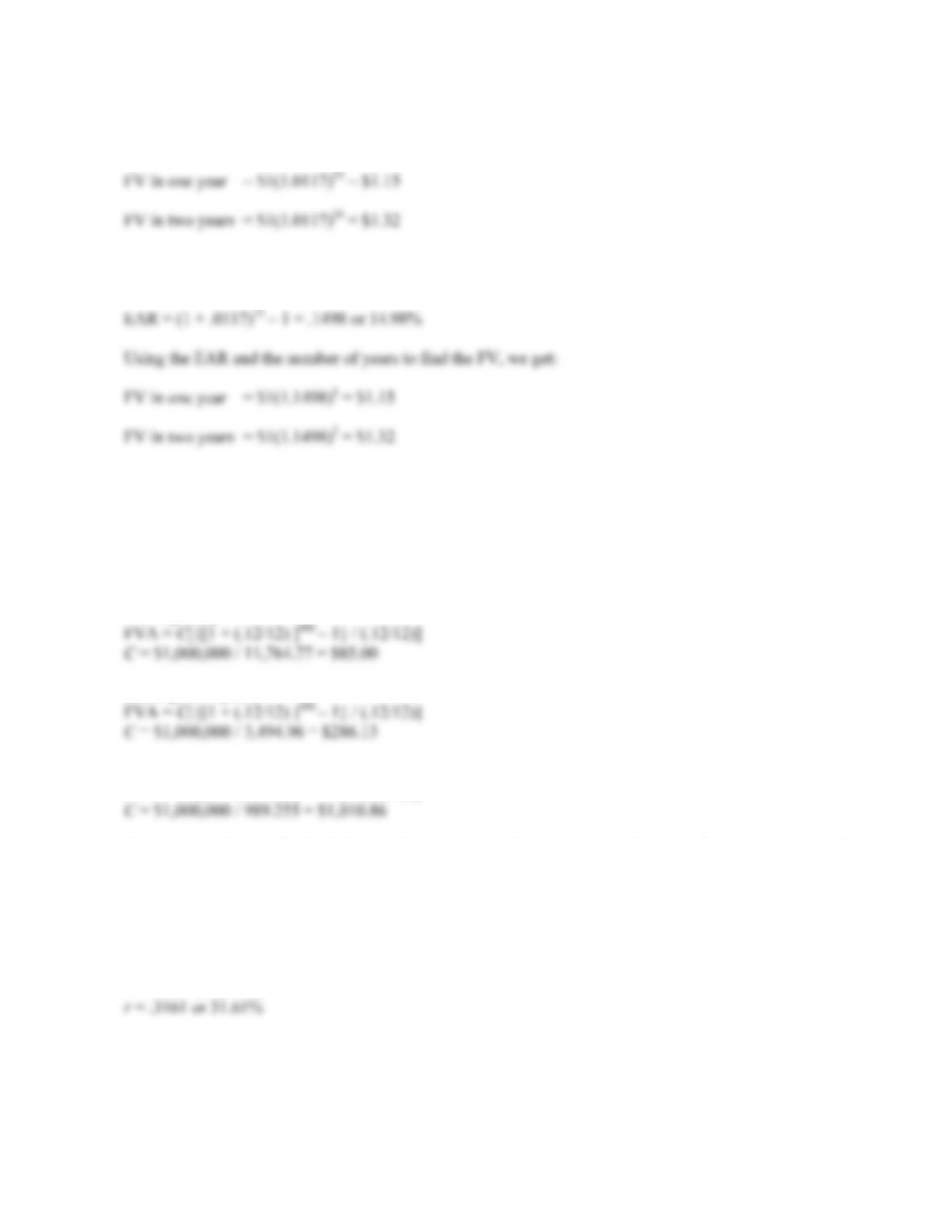B-78 SOLUTIONS
33. We need to find the FV of a lump sum in one year and two years. It is important that we use the
number of months in compounding since interest is compounded monthly in this case. So:
There is also another common alternative solution. We could find the EAR, and use the number of years as
our compounding periods. So we will find the EAR first:
Either method is correct and acceptable. We have simply made sure that the interest compounding period is
the same as the number of periods we use to calculate the FV.
34. Here we are finding the annuity payment necessary to achieve the same FV. The interest rate given is a 12
percent APR, with monthly deposits. We must make sure to use the number of months in the equation. So,
using the FVA equation:
Starting today:
Starting in 10 years:
Starting in 20 years:
FVA = C[{[1 + (.12/12) ]240 – 1} / (.12/12)]
Notice that a deposit for half the length of time, i.e. 20 years versus 40 years, does not mean that the
annuity payment is doubled. In this example, by reducing the savings period by one-half, the deposit
necessary to achieve the same ending value is about twelve times as large.
35. Since we are looking to quadruple our money, the PV and FV are irrelevant as long as the FV is three times
as large as the PV. The number of periods is four, the number of quarters per year. So:
FV = \$3 = \$1(1 + r)(12/3)